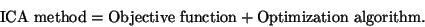Next: Multi-unit contrast functions Up: Objective (Contrast) Functions for Previous: Introduction

## Objective functions vs. algorithms

We draw here a distinction between the formulation of the objective function, and the algorithm used to optimize it. One might express this in the following 'equation':(12)

In the case of explicitly formulated objective functions, one can use any of the classical methods of optimization for optimizing the objective function, like (stochastic) gradient methods, Newton-like methods, etc. In some cases, however, the algorithm and the estimation principle may be difficult to separate.

The properties of the ICA method depend on both of the elements on the right-hand side of (12). In particular,

• the statistical properties (e.g., consistency, asymptotic variance, robustness) of the ICA method depend on the choice of the objective function, and
• the algorithmic properties (e.g., convergence speed, memory requirements, numerical stability) depend on the optimization algorithm.
These two classes of properties are independent in the sense that different optimization methods can be used to optimize a single objective function, and a single optimization method may be used to optimize different objective functions. In some cases, however, the distinction may not be so clear. In this Section, we shall treat only the choice of the objective function. The optimization of the objective function is treated in Section 5. Therefore, in this Section, we shall compare the objective functions mainly in terms of their statistical properties.Next: Multi-unit contrast functions Up: Objective (Contrast) Functions for Previous: Introduction
Aapo Hyvarinen
1999-04-23